##### Statistics: 1001 Practice Problems For Dummies (+ Free Online Practice)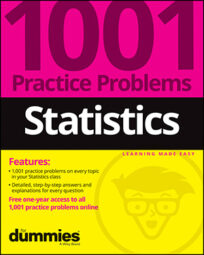Calculating a confidence interval for a population mean when the population standard deviation is known and the sample size is at least 30 involves the Z-distribution. When the population standard deviation is unknown, it involves a t-distribution. Calculate confidence intervals for population means in the following problems.

## Sample questions

1. In a random sample of 50 intramural basketball players at a large university, the average points per game was 8, with a standard deviation of 2.5 points and a 95% confidence level.

Which of the following statements is correct?

(A) With 95% confidence, the average points scored by all intramural basketball players is between 7.3 and 8.7 points.

(B) With 95% confidence, the average points scored by all intramural basketball players is between 7.7 and 8.4 points.

(C) With 95% confidence, the average points scored by all intramural basketball players is between 5.5 and 10.5 points.

(D) With 95% confidence, the average points scored by all intramural basketball players is between 7.2 and 8.8 points.

(E) With 95% confidence, the average points scored by all intramural basketball players is between 7.6 and 8.4 points.

Answer: A. With 95% confidence, the average points scored by all intramural basketball players is between 7.3 and 8.7 points.

Use the formula for finding the confidence interval for a population when the standard deviation is known: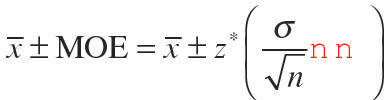where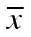is the sample mean,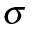is the population standard deviation, n is the sample size, and z* represents the appropriate z*-value from the standard normal distribution for your desired confidence level. The data has to come from a normal distribution, or n has to be large enough (a standard rule of thumb is at least 30 or so), for the central limit theorem to apply.

The z*-value is 1.96 for a two-tailed confidence interval with a confidence level of 95%.

Next, substitute the values into the formula: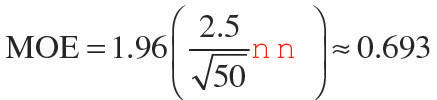The 95% confidence interval is 8 plus/minus 0.7 (rounded to the nearest tenth), or 7.3 to 8.7 points scored.

2. On the SAT Math test, a random sample of the scores of 100 students in a high school had a mean of 650.

The standard deviation for the population is 100. What is the confidence interval if 99% is the confidence level?

Answer: The 99% confidence interval for the average SAT math score for all students at the high school is between 624.2 and 678.8.

Use the formula for finding the confidence interval for a population when the standard deviation is known: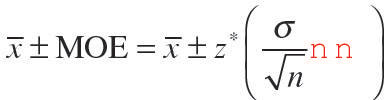whereis the sample mean,is the population standard deviation, n is the sample size, and z* represents the appropriate z*-value from the standard normal distribution for your desired confidence level. The data has to come from a normal distribution, or n has to be large enough (a standard rule of thumb is at least 30 or so), for the central limit theorem to apply.

The z*-value for a two-tailed confidence interval with a confidence level of 99% is 2.58.

Next, substitute the values into the formula: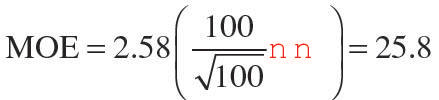The confidence interval is 650 plus/minus 25.8 (rounded to the nearest tenth), or 624.2 to 678.8.

3. An apple orchard harvested ten trees of apples. From a random sample of 50 apples, the mean weight of an apple was 7 ounces.

The population standard deviation is 1.5 ounces. What is the confidence interval if 99% is the confidence level?

Answer: The 99% confidence interval for the average weight of all apples from the ten trees is between 6.5 and 7.5 ounces.

Use the formula for finding the confidence interval for a population when the standard deviation is known: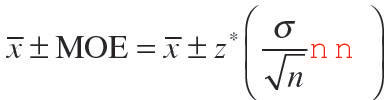whereis the sample mean,is the population standard deviation, n is the sample size, and z* represents the appropriate z*-value from the standard normal distribution for your desired confidence level. The data has to come from a normal distribution, or n has to be large enough (a standard rule of thumb is at least 30 or so), for the central limit theorem to apply.

The z*-value for a two-tailed confidence interval with a confidence level of 99% is 2.58.

Next, substitute the values into the formula: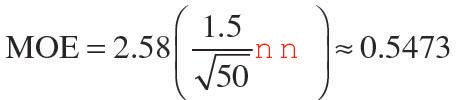The confidence interval is 7 plus/minus 0.5 (rounded to the nearest tenth), or 6.5 to 7.5 ounces.

If you need more practice on this and other topics from your statistics course, visit 1,001 Statistics Practice Problems For Dummies to purchase online access to 1,001 statistics practice problems! We can help you track your performance, see where you need to study, and create customized problem sets to master your stats skills.